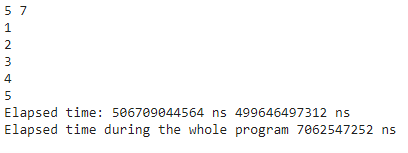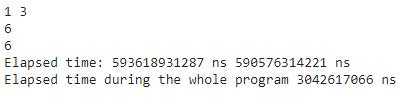# Python time.perf_counter_ns() Function

• Last Updated : 05 Nov, 2021

Python time.perf_counter_ns() function gives the integer value of time in nanoseconds.

Syntax:

`from time import perf_counter_ns`

We can find the elapsed time by using start and stop functions. We can also find the elapsed time during the whole program by subtracting stoptime and starttime

Example 1:

## Python3

 `# import  perf_counter_ns() ` `from` `time ``import` `perf_counter_ns ` ` `  `# integer input from user, 2 input in single line ` `n, m ``=` `map``(``int``, ``input``().split()) ` ` `  `# Start the stopwatch / counter ` `start ``=` `perf_counter_ns() ` ` `  `for` `i ``in` `range``(n): ` `    ``t ``=` `int``(``input``())  ``# user gave input n times ` `    ``if` `t ``%` `m ``=``=` `0``: ` `        ``print``(t) ` ` `  `# Stop the stopwatch / counter ` `stop ``=` `perf_counter_ns() ` ` `  `print``(``"Elapsed time:"``, stop, ``'ns'``, start, ``'ns'``) ` ` `  `print``(``"Elapsed time during the whole program"``, ` `      ``stop``-``start, ``'ns'``) `

Output:Example 2:

## Python3

 `# import  perf_counter_ns() ` `from` `time ``import` `perf_counter_ns ` ` `  `# integer input from user, 2 input in single line ` `n, m ``=` `map``(``int``, ``input``().split()) ` ` `  `# Start the stopwatch / counter ` `start ``=` `perf_counter_ns() ` ` `  `for` `i ``in` `range``(n): ` `    ``t ``=` `int``(``input``())  ``# user gave input n times ` `    ``if` `t ``%` `m ``=``=` `0``: ` `        ``print``(t) ` ` `  `# Stop the stopwatch / counter ` `stop ``=` `perf_counter_ns() ` ` `  `print``(``"Elapsed time:"``, stop, ``'ns'``, start, ``'ns'``) ` ` `  `print``(``"Elapsed time during the whole program"``, ` `      ``stop``-``start, ``'ns'``) `

Output:My Personal Notes arrow_drop_up
Recommended Articles
Page :